# RD Sharma Solutions Class 12 Binomial Distribution Exercise 33.2

RD Sharma Solutions for Class 12 Maths Exercise 33.2 Chapter 33 Binomial Distribution are provided here. This exercise mainly deals with Mean and Variance. To cover the entire syllabus in Maths, the RD Sharma Solutions for Class 12 Maths Chapter 33 is an essential material as it offers a wide range of questions that test the students’ understanding of concepts.

## Download PDF of Rd Sharma Solution for Class 12 Maths Chapter 33 Exercise 2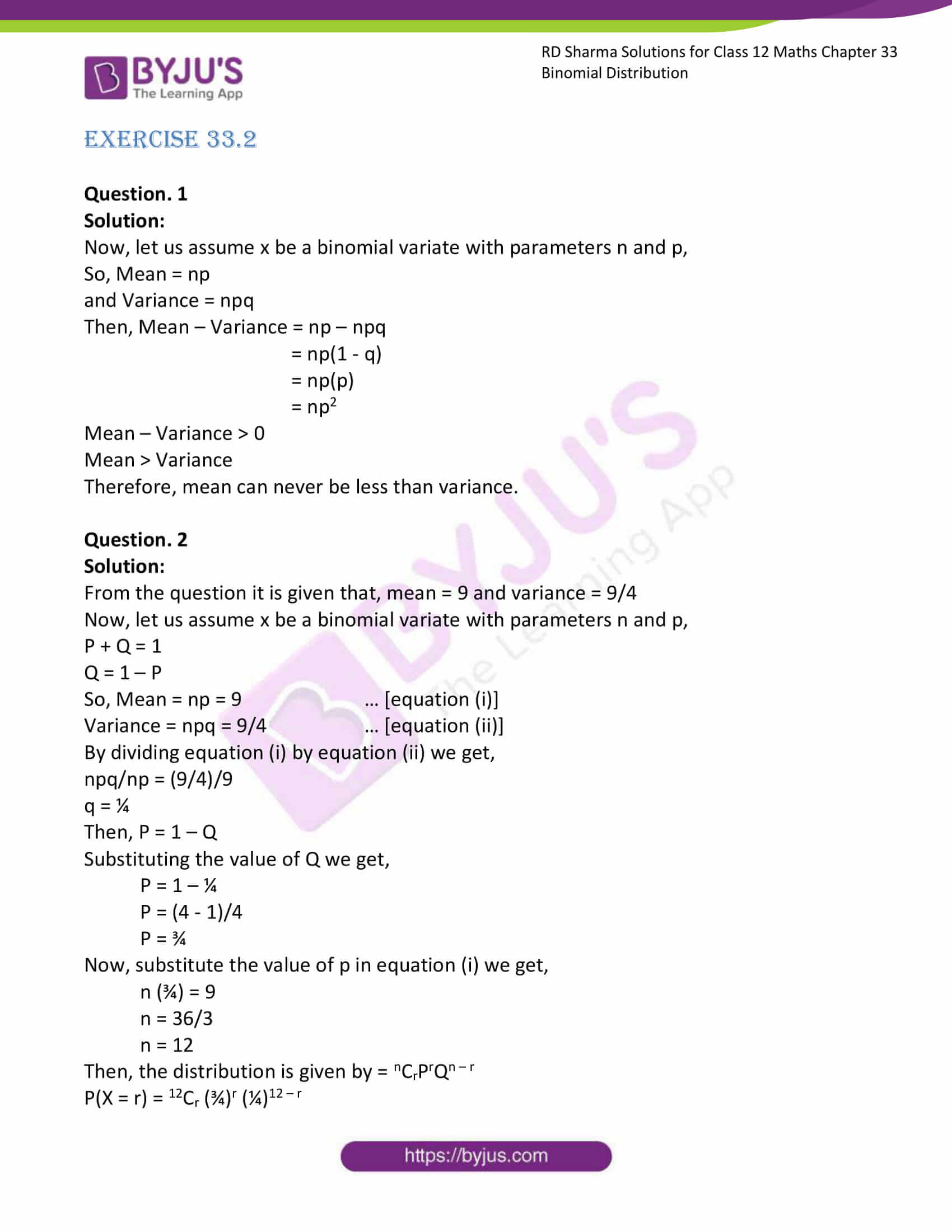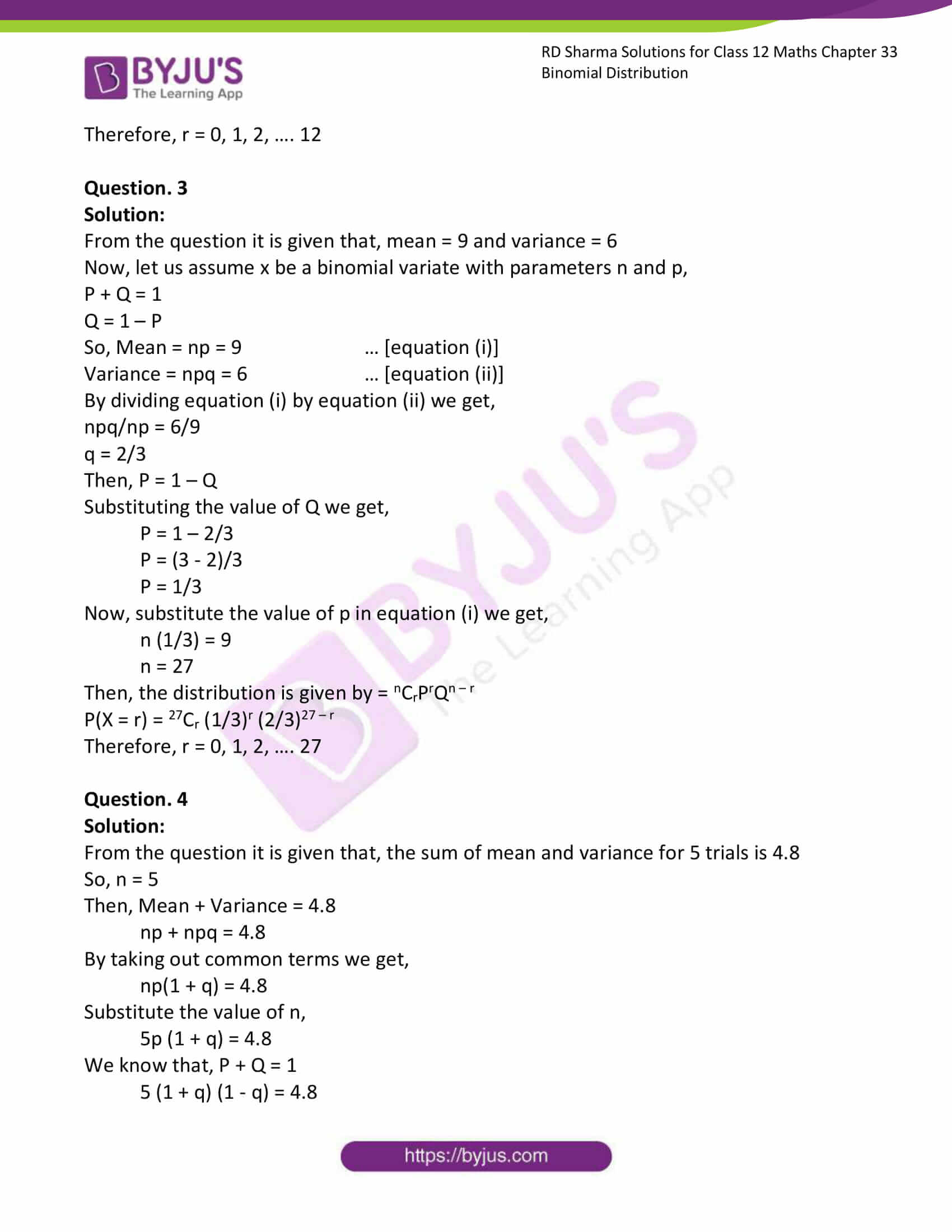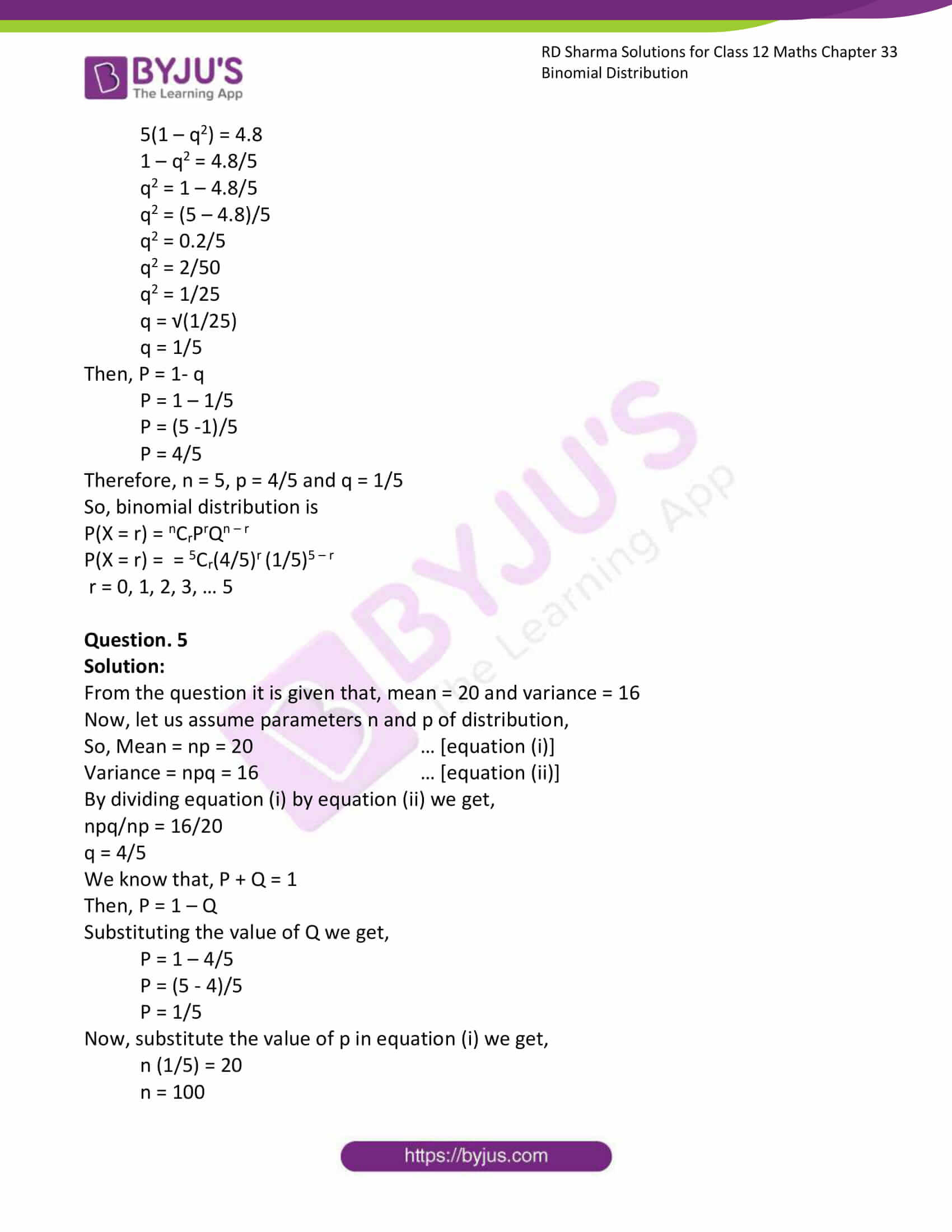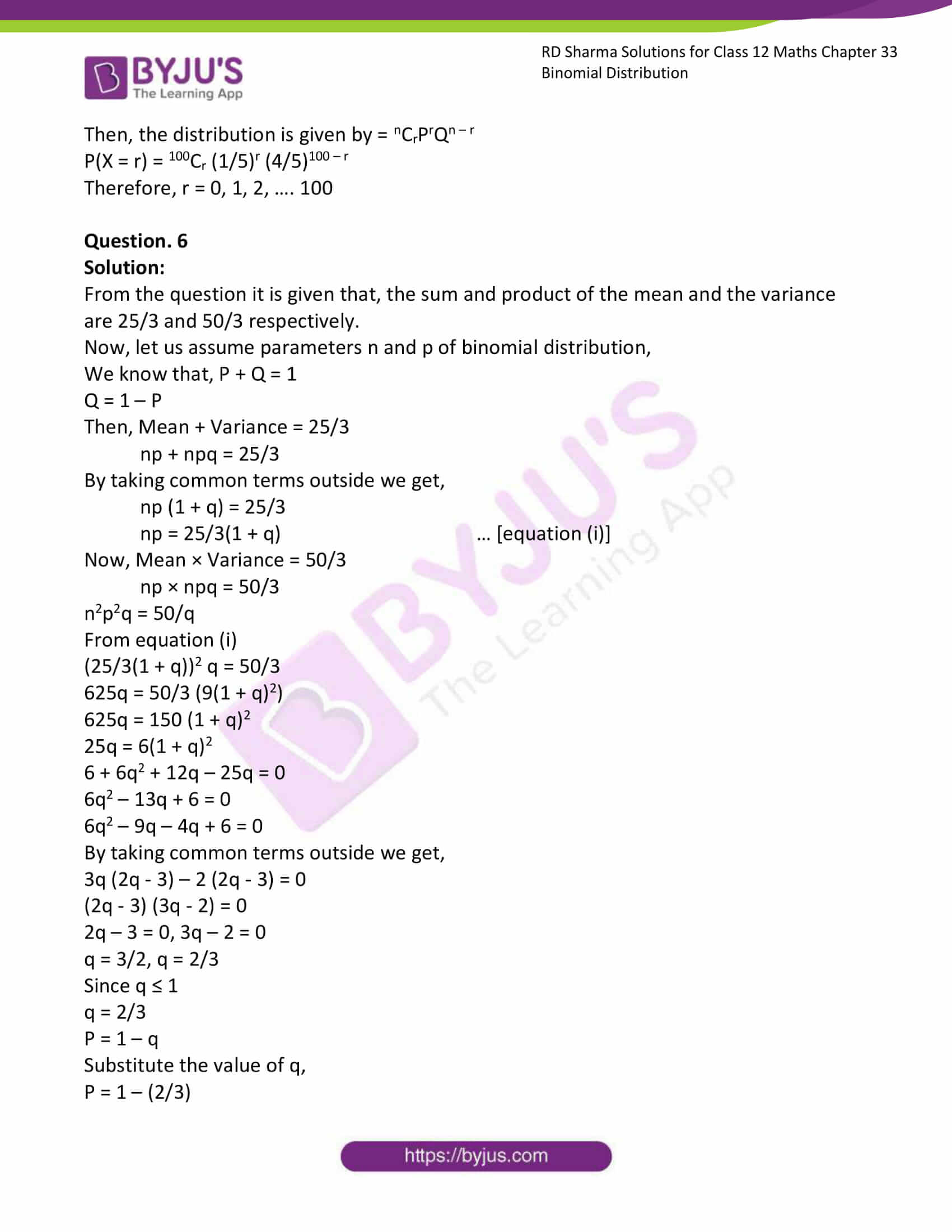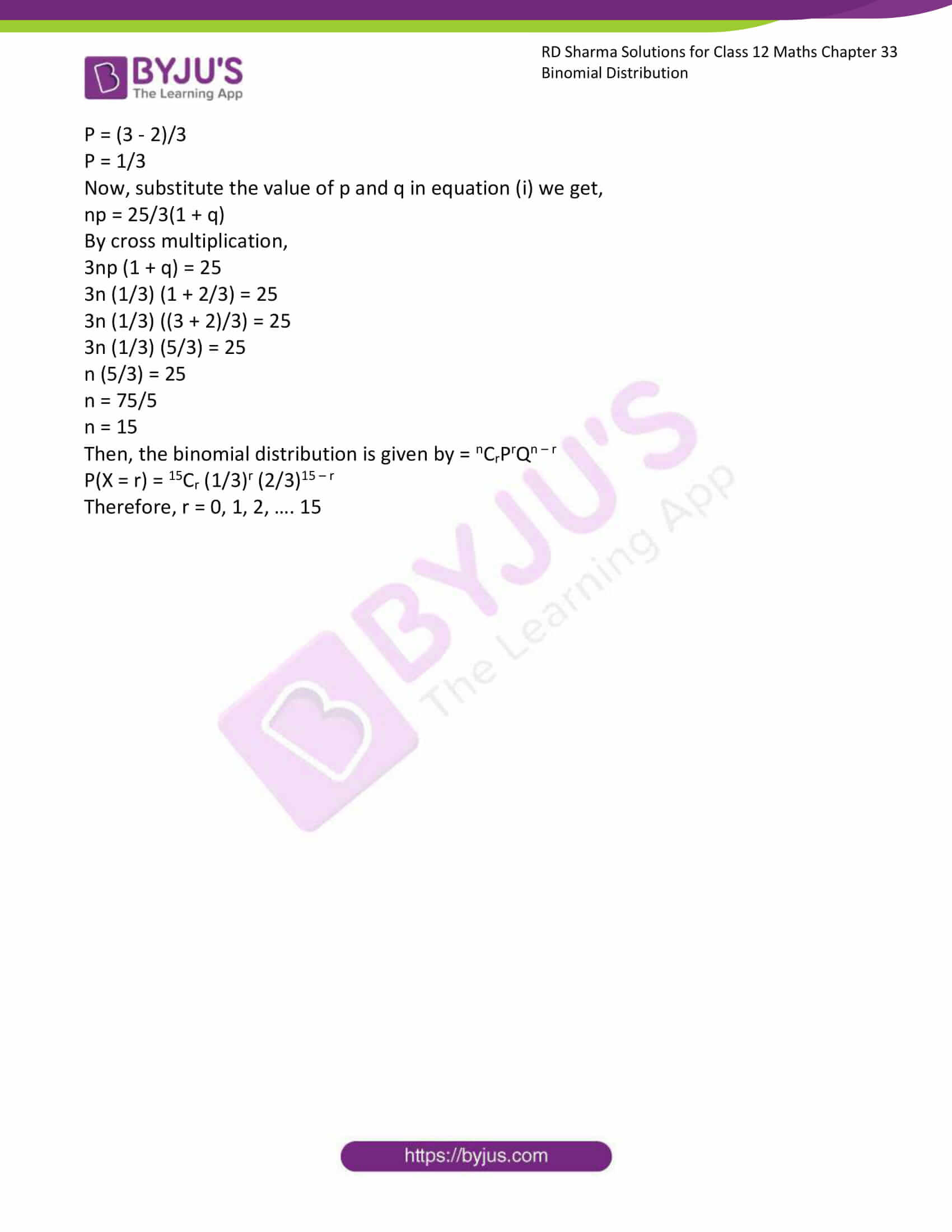### Access Answers for Rd Sharma Solution Class 12 Maths Chapter 33 Exercise 2

EXERCISE 33.2

Question. 1

Solution:

Now, let us assume x be a binomial variate with parameters n and p,

So, Mean = np

and Variance = npq

Then, Mean – Variance = np – npq

= np(1 – q)

= np(p)

= np2

Mean – Variance > 0

Mean > Variance

Therefore, mean can never be less than variance.

Question. 2

Solution:

From the question it is given that, mean = 9 and variance = 9/4

Now, let us assume x be a binomial variate with parameters n and p,

P + Q = 1

Q = 1 – P

So, Mean = np = 9 … [equation (i)]

Variance = npq = 9/4 … [equation (ii)]

By dividing equation (i) by equation (ii) we get,

npq/np = (9/4)/9

q = ¼

Then, P = 1 – Q

Substituting the value of Q we get,

P = 1 – ¼

P = (4 – 1)/4

P = ¾

Now, substitute the value of p in equation (i) we get,

n (¾) = 9

n = 36/3

n = 12

Then, the distribution is given by = nCrPrQn – r

P(X = r) = 12Cr (¾)r (¼)12 – r

Therefore, r = 0, 1, 2, …. 12

Question. 3

Solution:

From the question it is given that, mean = 9 and variance = 6

Now, let us assume x be a binomial variate with parameters n and p,

P + Q = 1

Q = 1 – P

So, Mean = np = 9 … [equation (i)]

Variance = npq = 6 … [equation (ii)]

By dividing equation (i) by equation (ii) we get,

npq/np = 6/9

q = 2/3

Then, P = 1 – Q

Substituting the value of Q we get,

P = 1 – 2/3

P = (3 – 2)/3

P = 1/3

Now, substitute the value of p in equation (i) we get,

n (1/3) = 9

n = 27

Then, the distribution is given by = nCrPrQn – r

P(X = r) = 27Cr (1/3)r (2/3)27 – r

Therefore, r = 0, 1, 2, …. 27

Question. 4

Solution:

From the question it is given that, the sum of mean and variance for 5 trials is 4.8

So, n = 5

Then, Mean + Variance = 4.8

np + npq = 4.8

By taking out common terms we get,

np(1 + q) = 4.8

Substitute the value of n,

5p (1 + q) = 4.8

We know that, P + Q = 1

5 (1 + q) (1 – q) = 4.8

5(1 – q2) = 4.8

1 – q2 = 4.8/5

q2 = 1 – 4.8/5

q2 = (5 – 4.8)/5

q2 = 0.2/5

q2 = 2/50

q2 = 1/25

q = √(1/25)

q = 1/5

Then, P = 1- q

P = 1 – 1/5

P = (5 -1)/5

P = 4/5

Therefore, n = 5, p = 4/5 and q = 1/5

So, binomial distribution is

P(X = r) = nCrPrQn – r

P(X = r) = = 5Cr(4/5)r (1/5)5 – r

r = 0, 1, 2, 3, … 5

Question. 5

Solution:

From the question it is given that, mean = 20 and variance = 16

Now, let us assume parameters n and p of distribution,

So, Mean = np = 20 … [equation (i)]

Variance = npq = 16 … [equation (ii)]

By dividing equation (i) by equation (ii) we get,

npq/np = 16/20

q = 4/5

We know that, P + Q = 1

Then, P = 1 – Q

Substituting the value of Q we get,

P = 1 – 4/5

P = (5 – 4)/5

P = 1/5

Now, substitute the value of p in equation (i) we get,

n (1/5) = 20

n = 100

Then, the distribution is given by = nCrPrQn – r

P(X = r) = 100Cr (1/5)r (4/5)100 – r

Therefore, r = 0, 1, 2, …. 100

Question. 6

Solution:

From the question it is given that, the sum and product of the mean and the variance are 25/3 and 50/3 respectively.

Now, let us assume parameters n and p of binomial distribution,

We know that, P + Q = 1

Q = 1 – P

Then, Mean + Variance = 25/3

np + npq = 25/3

By taking common terms outside we get,

np (1 + q) = 25/3

np = 25/3(1 + q) … [equation (i)]

Now, Mean × Variance = 50/3

np × npq = 50/3

n2p2q = 50/q

From equation (i)

(25/3(1 + q))2 q = 50/3

625q = 50/3 (9(1 + q)2)

625q = 150 (1 + q)2

25q = 6(1 + q)2

6 + 6q2 + 12q – 25q = 0

6q2 – 13q + 6 = 0

6q2 – 9q – 4q + 6 = 0

By taking common terms outside we get,

3q (2q – 3) – 2 (2q – 3) = 0

(2q – 3) (3q – 2) = 0

2q – 3 = 0, 3q – 2 = 0

q = 3/2, q = 2/3

Since q ≤ 1

q = 2/3

P = 1 – q

Substitute the value of q,

P = 1 – (2/3)

P = (3 – 2)/3

P = 1/3

Now, substitute the value of p and q in equation (i) we get,

np = 25/3(1 + q)

By cross multiplication,

3np (1 + q) = 25

3n (1/3) (1 + 2/3) = 25

3n (1/3) ((3 + 2)/3) = 25

3n (1/3) (5/3) = 25

n (5/3) = 25

n = 75/5

n = 15

Then, the binomial distribution is given by = nCrPrQn – r

P(X = r) = 15Cr (1/3)r (2/3)15 – r

Therefore, r = 0, 1, 2, …. 15